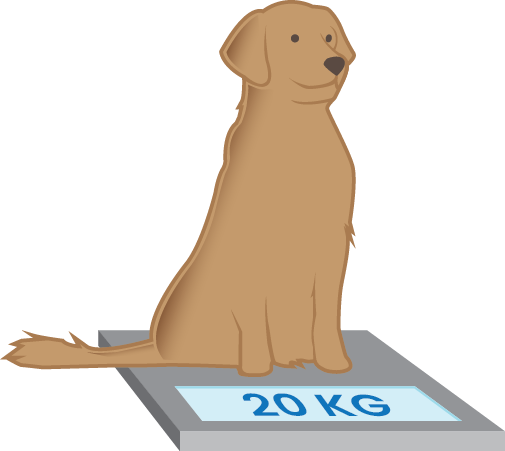# What Are the Metric Units for Weight?

This entry uses the metric system. For the imperial system click here.

When you want do describe how heavy something is, you are talking about weight. What’s more difficult to lift: A fork or a TV? How many forks do you need before the weight of all the forks is larger than the weight of the TV?

If you have two things and wonder what weighs the most, you can bring them to the playground and lay them on each side of a 2-way seesaw. The side of the seesaw that falls to the ground has the object weighing the most on top of it.Weight is measured in kilograms, which is abbreviated to “kg”. A full carton of milk weighs about one kilogram. That means that if the neighbor’s dog weighs $20$ kilograms, it will weigh as much as $20$ full cartons of milk. You can verify this with the seesaw at the nearest playground.If you’re standing on top of a bathroom scale, it will tell you how many kilograms you weigh. This weight can be used instead of the seesaw, so that you don’t have to walk all the way to the playground every time you want to weigh something.You just learned that the bathroom scale you are standing on tells you how much you weigh in kilograms. Kilo is Greek and means thousand. That means that one kilogram is the same as a thousand grams (g). In the same way that we split distance into different units, we split gram into different units. You focus on kilograms for most items in your everyday life. However, for baking or cooking where you need small amounts of something, you often need to switch to smaller units. The division from kilograms into smaller units is what we will look at next.Hectograms (hg) is $100$ grams. Hecto- means hundred. For that reason, one hectogram is the same as $100$ grams.

Decagram (dag) is $10$ grams. Deda- means ten. For that reason, one decagram is the same as $10$ grams.

Decigram (dg) is $0.1$ grams. Deci- means tenth. For that reason, one decigram is the same as $0.1$ grams.

Centigram (cg) is $0.01$ grams. Centi- means hundredth. For that reason, one centigram is the same as $0.01$ grams.

Milligram (mg) is $0.001$ grams. Milli- means thousandth. For that reason, one milligram is the same as $0.001$ grams.If you want to weigh something heavy, you can use what is called a tonne. One tonne is one thousand kilograms, i.e. $1\phantom{\rule{0.17em}{0ex}}000\phantom{\rule{0.17em}{0ex}}000$ grams. An adult hippopotamus weighs even more than that!Example 1

Kilogram to hectogram: $\begin{array}{llll}\hfill 50\phantom{\rule{0.17em}{0ex}}\text{kg}& =\left(50\cdot 10\right)\phantom{\rule{0.33em}{0ex}}\text{hg}\phantom{\rule{2em}{0ex}}& \hfill & \phantom{\rule{2em}{0ex}}\\ \hfill & =500\phantom{\rule{0.17em}{0ex}}\text{hg}\phantom{\rule{2em}{0ex}}& \hfill & \phantom{\rule{2em}{0ex}}\end{array}$

Hectogram to kilogram: $\begin{array}{llll}\hfill 500\phantom{\rule{0.17em}{0ex}}\text{hg}& =\left(500÷10\right)\phantom{\rule{0.33em}{0ex}}\text{kg}\phantom{\rule{2em}{0ex}}& \hfill & \phantom{\rule{2em}{0ex}}\\ \hfill & =50\phantom{\rule{0.17em}{0ex}}\text{kg}\phantom{\rule{2em}{0ex}}& \hfill & \phantom{\rule{2em}{0ex}}\end{array}$

Hectogram to gram: $\begin{array}{llll}\hfill 2\phantom{\rule{0.17em}{0ex}}\text{hg}& =\left(2\cdot 10\right)\phantom{\rule{0.33em}{0ex}}\text{dag}\phantom{\rule{2em}{0ex}}& \hfill & \phantom{\rule{2em}{0ex}}\\ \hfill & =20\phantom{\rule{0.17em}{0ex}}\text{dag}\phantom{\rule{2em}{0ex}}& \hfill & \phantom{\rule{2em}{0ex}}\\ \hfill & =\left(20\cdot 10\right)\phantom{\rule{0.33em}{0ex}}\text{g}\phantom{\rule{2em}{0ex}}& \hfill & \phantom{\rule{2em}{0ex}}\\ \hfill & =200\phantom{\rule{0.17em}{0ex}}\text{g}\phantom{\rule{2em}{0ex}}& \hfill & \phantom{\rule{2em}{0ex}}\end{array}$

Gram to hectogram: $\begin{array}{llll}\hfill 200\phantom{\rule{0.17em}{0ex}}\text{g}& =\left(200÷10\right)\phantom{\rule{0.33em}{0ex}}\text{dag}\phantom{\rule{2em}{0ex}}& \hfill & \phantom{\rule{2em}{0ex}}\\ \hfill & =20\phantom{\rule{0.17em}{0ex}}\text{dag}\phantom{\rule{2em}{0ex}}& \hfill & \phantom{\rule{2em}{0ex}}\\ \hfill & =\left(20÷10\right)\phantom{\rule{0.33em}{0ex}}\text{hg}\phantom{\rule{2em}{0ex}}& \hfill & \phantom{\rule{2em}{0ex}}\\ \hfill & =2\phantom{\rule{0.17em}{0ex}}\text{hg}\phantom{\rule{2em}{0ex}}& \hfill & \phantom{\rule{2em}{0ex}}\end{array}$

Gram to milligram: $\begin{array}{llll}\hfill 4\phantom{\rule{0.17em}{0ex}}\text{g}& =\left(4\cdot 10\right)\phantom{\rule{0.33em}{0ex}}\text{dg}\phantom{\rule{2em}{0ex}}& \hfill & \phantom{\rule{2em}{0ex}}\\ \hfill & =40\phantom{\rule{0.17em}{0ex}}\text{dg}\phantom{\rule{2em}{0ex}}& \hfill & \phantom{\rule{2em}{0ex}}\\ \hfill & =\left(40\cdot 10\right)\phantom{\rule{0.33em}{0ex}}\text{cg}\phantom{\rule{2em}{0ex}}& \hfill & \phantom{\rule{2em}{0ex}}\\ \hfill & =400\phantom{\rule{0.17em}{0ex}}\text{cg}\phantom{\rule{2em}{0ex}}& \hfill & \phantom{\rule{2em}{0ex}}\\ \hfill & =\left(400\cdot 10\right)\phantom{\rule{0.33em}{0ex}}\text{mg}\phantom{\rule{2em}{0ex}}& \hfill & \phantom{\rule{2em}{0ex}}\\ \hfill & =4000\phantom{\rule{0.17em}{0ex}}\text{mg}\phantom{\rule{2em}{0ex}}& \hfill & \phantom{\rule{2em}{0ex}}\end{array}$

Milligram to gram: $\begin{array}{llll}\hfill 4000\phantom{\rule{0.17em}{0ex}}\text{mg}& =\left(4000÷10\right)\phantom{\rule{0.33em}{0ex}}\text{cg}\phantom{\rule{2em}{0ex}}& \hfill & \phantom{\rule{2em}{0ex}}\\ \hfill & =400\phantom{\rule{0.17em}{0ex}}\text{cg}\phantom{\rule{2em}{0ex}}& \hfill & \phantom{\rule{2em}{0ex}}\\ \hfill & =\left(400÷10\right)\phantom{\rule{0.33em}{0ex}}\text{dg}\phantom{\rule{2em}{0ex}}& \hfill & \phantom{\rule{2em}{0ex}}\\ \hfill & =40\phantom{\rule{0.17em}{0ex}}\text{dg}\phantom{\rule{2em}{0ex}}& \hfill & \phantom{\rule{2em}{0ex}}\\ \hfill & =\left(40÷10\right)\phantom{\rule{0.33em}{0ex}}\text{g}\phantom{\rule{2em}{0ex}}& \hfill & \phantom{\rule{2em}{0ex}}\\ \hfill & =4\phantom{\rule{0.17em}{0ex}}\text{g}\phantom{\rule{2em}{0ex}}& \hfill & \phantom{\rule{2em}{0ex}}\end{array}$

Math Vault

Would you like to solve exercises about weight? Try Math Vault!